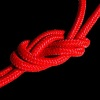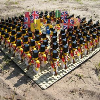# Special Numbers

In some senses, all numbers are special, though some are perhaps given more attention than others...

The Special Numbers pathway on wild.maths.org invites students to take a closer look at some familiar categories of number and operations, and perhaps discover that there's a lot more to averages, fractions and square numbers than they might have initially thought.

The collection of related NRICH tasks below are ideal for teachers who want to promote creativity in the classroom. They are designed for classroom use, with accompanying Teachers' Notes and Resources.### M, M and M

##### Age 11 to 14 Challenge Level:

If you are given the mean, median and mode of five positive whole numbers, can you find the numbers?### Keep it Simple

##### Age 11 to 14 Challenge Level:

Can all unit fractions be written as the sum of two unit fractions?### Unequal Averages

##### Age 11 to 14 Challenge Level:

Play around with sets of five numbers and see what you can discover about different types of average...##### Age 11 to 14 Challenge Level:

Powers of numbers behave in surprising ways. Take a look at some of these and try to explain why they are true.### Egyptian Fractions

##### Age 11 to 14 Challenge Level:

The Egyptians expressed all fractions as the sum of different unit fractions. Here is a chance to explore how they could have written different fractions.### Peaches Today, Peaches Tomorrow...

##### Age 11 to 14 Challenge Level:

A monkey with peaches, keeps a fraction of them each day, gives the rest away, and then eats one. How long can his peaches last?### Twisting and Turning

##### Age 11 to 14 Challenge Level:

Take a look at the video and try to find a sequence of moves that will untangle the ropes.### The Greedy Algorithm

##### Age 11 to 14 Challenge Level:

The Egyptians expressed all fractions as the sum of different unit fractions. The Greedy Algorithm might provide us with an efficient way of doing this.### Searching for Mean(ing)

##### Age 11 to 16 Challenge Level:

If you have a large supply of 3kg and 8kg weights, how many of each would you need for the average (mean) of the weights to be 6kg?### Wipeout

##### Age 11 to 16 Challenge Level:

Can you do a little mathematical detective work to figure out which number has been wiped out?### Big Powers

##### Age 11 to 16 Challenge Level:

Three people chose this as a favourite problem. It is the sort of problem that needs thinking time - but once the connection is made it gives access to many similar ideas.### Hollow Squares

##### Age 14 to 16 Challenge Level:

Which armies can be arranged in hollow square fighting formations?### Generating Triples

##### Age 14 to 16 Challenge Level:

Sets of integers like 3, 4, 5 are called Pythagorean Triples, because they could be the lengths of the sides of a right-angled triangle. Can you find any more?### Plus Minus

##### Age 14 to 16 Challenge Level:

Can you explain the surprising results Jo found when she calculated the difference between square numbers?### Fair Shares?

##### Age 14 to 16 Challenge Level:

A mother wants to share a sum of money by giving each of her children in turn a lump sum plus a fraction of the remainder. How can she do this in order to share the money out equally?### What's Possible?

##### Age 14 to 16 Challenge Level:

Many numbers can be expressed as the difference of two perfect squares. What do you notice about the numbers you CANNOT make?